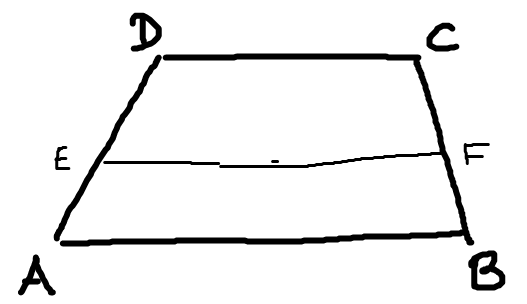# Résolution de problèmes algorithmiques

Divide a trapezoid into two halfs of same area.### Input

A Trapezoid A-B-C-D

But only the edge lengths AB, BC, CD, DA are given.

### Output

Points E,F such that EF is parallel to AB (and hence CD), and divides the trapezoid into two halfs of same area.

### Solution

We aim for a value 0≤x≤1, such that when x=0, E=A, F=B and for x=1, E=D, F=C. More precisely E is a point on the segment AD at a position defined by x, and similarly is F on the segment BC.

Then EF = (1-x)AB + xDC.

The condition that both areas are the same gives:

((1-x)AB + xDC + AB)x h/2 = ((1-x)AB + xDC)(1-x) h/2


where h is the height of the trapezoid. We obtain

2(DC - AB) x^2 + 4AB - AB - DC = 0


and solve it for x.